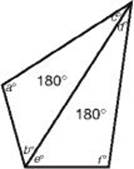﻿ ﻿THE ANGLES OF A POLYGON - Quadrilaterals and Other Polygons - PLANE GEOMETRY - SAT SUBJECT TEST MATH LEVEL 1

## PLANE GEOMETRY## CHAPTER 10Quadrilaterals and Other Polygons• The Angles of a Polygon

• Perimeter and Area of Quadrilaterals

• Exercises

A polygon is a closed geometric figure made up of line segments. The line segments are called sides, and the endpoints of the line segments are called vertices (each one is called a vertex). Line segments inside the polygon drawn from one vertex to another are called diagonals.

Three-sided polygons, called triangles, were discussed in Chapter 9. Although in this section our main focus will be on four-sided polygons, which are called quadrilaterals, we will discuss other polygons as well. There are special names for many polygons with more than four sides. The ones you need to know for the Math 1 test are given in the following chart.A regular polygon is a polygon in which all the sides have the same length and all the angles have the same measure. A regular three-sided polygon is an equilateral triangle, and, as we shall see, a regular quadrilateral is a square. Pictured below are a regular pentagon, regular hexagon, and regular octagon.pentagonhexagonoctagon

Remember

In a regular polygon, all the angles are congruent and all the sides are congruent.

### THE ANGLES OF A POLYGON

A diagonal of a quadrilateral divides it into two triangles. Since the sum of the measures of the three angles in each of the triangles is 180°, the sum of the measures of the angles in the quadrilateral is 360°.a + b + c = 180 and d + e + f = 180
a + (b + e ) + (c + d ) + f = 360

Key Fact I1

In any quadrilateral, the sum of the measures of the four angles is 360°.

Similarly, any polygon can be divided into triangles by drawing in all of the diagonals emanating from one vertex.pentagonhexagonoctagon

Notice that a five-sided polygon can be divided into three triangles, and a six-sided polygon can be divided into four triangles. In general, an n-sided polygon can be divided into (n – 2) triangles, which leads to KEY FACT I2.

Key Fact I2

The sum of the measures of the n angles in a polygon with n sides is (n2)180°.

EXAMPLE 1: To find the measure of each angle of a regular octagon, first use KEY FACT I2 to get that the sum of all eight angles is (8 – 2)180° = 6180° = 1,080°. Then since in a regular octagon all eight angles have the same measure, the measure of each one is 1,080°8 = 135°.

An exterior angle of a polygon is formed by extending a side. Surprisingly, in all polygons, the sum of the measures of the exterior angles is the same.

Key Fact I3

In any polygon, the sum of the measures of the exterior angles, taking one at each vertex, is 360°.x + y + z = 360a + b + c + d + e = 360

EXAMPLE 2: KEY FACT I3 gives us an alternative method of calculating the measure of each angle in a regular polygon. In Example 1 we used KEY FACT I2 to find the measure of each angle in a regular octagon. By KEY FACT I3, the sum of the measures of the eight exterior angles of any octagon is 360°. As a result, in a regular octagon, the measure of each exterior angle is 360°8 = 45°. Therefore, the measure of each interior angle is 180° – 45° = 135°.

﻿